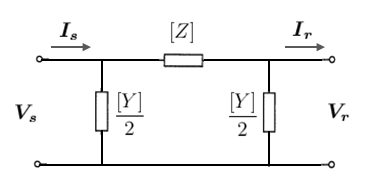# Multi-conductor Line Models

(diff) ← Older revision | Latest revision (diff) | Newer revision → (diff)
Jump to navigation Jump to search

## IntroductionFigure 1. Three-phase, single-circuit tower line (image courtesy of Wikipedia)

Single-phase equivalent line models (or positive-sequence line models) can be used quite accurately in three-phase systems when the system is balanced and the lines are perfectly transposed. However, when unbalanced systems or untransposed lines are being studied, these models break down and a full three-phase multi-conductor model is necessary.

Consider the three-phase, single circuit tower line in Figure 1, which shows three phase conductors and an earth wire. A segmental length of this multi-conductor system can be represented by the equivalent circuit in Figure 2 below:

We can see that in a multi-conductor system, there is mutual coupling between the phase conductors (a, b and c), represented by the shunt inductances and capacitances. In the single-phase equivalent line model, these mutual couplings are ignored. Note that there could also be resistive coupling between phases, but is not shown in Figure 2 since resistive coupling is normally assumed to be negligible in overhead lines (i.e. shunt conductances = 0).

Recall that in the single-phase equivalent line model, we have single complex quantities for the series impedance ${\boldsymbol {Z}}=R+jX_{L}\,$and shunt admittance ${\boldsymbol {Y}}=G+jB\,$of the line. But in the multi-conductor line, these single complex quantities are replaced by $n\times n$matrices, where n is the number of conductors in the system.

For example, the four conductor system in Figure 2 has the $4\times 4$impedance matrix:

$[Z]=\left[{\begin{matrix}Z_{aa}&Z_{ab}&Z_{ac}&|&Z_{ae}\\Z_{ba}&Z_{bb}&Z_{bc}&|&Z_{be}\\Z_{ca}&Z_{cb}&Z_{cc}&|&Z_{ce}\\--&--&--&|&--\\Z_{ea}&Z_{eb}&Z_{ec}&|&Z_{ee}\end{matrix}}\right]\,$And the $4\times 4$admittance matrix:

$[Y]=\left[{\begin{matrix}Y_{aa}&Y_{ab}&Y_{ac}&|&Y_{ae}\\Y_{ba}&Y_{bb}&Y_{bc}&|&Y_{be}\\Y_{ca}&Y_{cb}&Y_{cc}&|&Y_{ce}\\--&--&--&|&--\\Y_{ea}&Y_{eb}&Y_{ec}&|&Y_{ee}\end{matrix}}\right]\,$Since we assume that under normal conditions, the earth wire is at zero potential (i.e. no voltage between the earth wire and neutral), we can use the Kron reduction to reduce the impedance and admittance matrices from $n\times n$to $3\times 3$matrices, i.e.

$[Z']=\left[{\begin{matrix}Z_{aa}'&Z_{ab}'&Z_{ac}'\\Z_{ba}'&Z_{bb}'&Z_{bc}'\\Z_{ca}'&Z_{cb}'&Z_{cc}'\end{matrix}}\right]\,$$[Y']=\left[{\begin{matrix}Y_{aa}'&Y_{ab}'&Y_{ac}'\\Y_{ba}'&Y_{bb}'&Y_{bc}'\\Y_{ca}'&Y_{cb}'&Y_{cc}'\end{matrix}}\right]\,$We call these matrices the Kron reduced impedance and admittance matrices.

## Nominal $\pi$LineFigure 3. Multi-conductor nominal $\pi$line model

The multi-conductor nominal $\pi$line model shown in Figure 3 is a direct substitution of the $1\times 1$complex parameters in the single-phase nominal $\pi$Line with $n\times n$matrices.

If we consider the Kron reduced case, [Z] and [Y] are $3\times 3$matrices as described in the previous section. The voltages and currents are $3\times 1$complex vectors of the form:

${\boldsymbol {V_{s}}}=\left[{\begin{matrix}{\boldsymbol {V_{s,a}}}\\{\boldsymbol {V_{s,b}}}\\{\boldsymbol {V_{s,c}}}\end{matrix}}\right]\,$, ${\boldsymbol {I_{s}}}=\left[{\begin{matrix}{\boldsymbol {I_{s,a}}}\\{\boldsymbol {I_{s,b}}}\\{\boldsymbol {I_{s,c}}}\end{matrix}}\right]\,$, ${\boldsymbol {V_{r}}}=\left[{\begin{matrix}{\boldsymbol {V_{r,a}}}\\{\boldsymbol {V_{r,b}}}\\{\boldsymbol {V_{r,c}}}\end{matrix}}\right]\,$and ${\boldsymbol {I_{r}}}=\left[{\begin{matrix}{\boldsymbol {I_{r,a}}}\\{\boldsymbol {I_{r,b}}}\\{\boldsymbol {I_{r,c}}}\end{matrix}}\right]\,$The ABCD parameters of the multi-conductor nominal $\pi$line are:

$\left[{\begin{matrix}{\boldsymbol {V_{s}}}\\\\{\boldsymbol {I_{s}}}\end{matrix}}\right]=\left[{\begin{matrix}\left(I+{\frac {[Z][Y]}{2}}\right)&[Z]\\\\Y\left(I+{\frac {[Z][Y]}{4}}\right)&\left(I+{\frac {[Z][Y]}{2}}\right)\end{matrix}}\right]\left[{\begin{matrix}{\boldsymbol {V_{r}}}\\\\{\boldsymbol {I_{r}}}\end{matrix}}\right]\,$## Distributed Parameter Line

Refer to the distributed parameter model article for the detailed derivation of the model.

In the single-phase line model, we saw that it was possible to represent a distributed parameter line model using the same equivalent circuit as the nominal $\pi$model, but with adjusted line parameters Z and Y. This was called the equivalent $\pi$model and the adjusted parameters could be calculated as follows:

${\boldsymbol {Z'}}={\boldsymbol {Z}}_{c}\sinh({\boldsymbol {\gamma }}l)$${\frac {\boldsymbol {Y'}}{2}}={\frac {1}{{\boldsymbol {Z}}_{c}}}\tanh \left({\frac {{\boldsymbol {\gamma }}l}{2}}\right)$Where $\gamma ={\sqrt {\boldsymbol {zy}}}$is the propagation constant $m^{-1}$However in the multi-conductor line, the propagation constant would be a matrix of the form:

$[\gamma ]=\left([Z][Y]\right)^{\frac {1}{2}}$There is no straightforward method for calculating the hyperbolic sine and tangent functions of a matrix (the hyperbolic functions can be expanded as a Taylor series, but this is still not particularly easy to compute). This led to the development of the modal transformation, which is a method for decoupling the phases of the impedance and admittance matrices.

The ABCD parameters of the multi-conductor distributed parameter line are (in modal form):

$\left[{\begin{matrix}{\boldsymbol {V_{s}'}}\\\\{\boldsymbol {I_{s}'}}\end{matrix}}\right]=\left[{\begin{matrix}\left[\cosh {(\gamma l)}\right]&\left[{\boldsymbol {Z_{c}}}\sinh {(\gamma l)}\right]\\\\\left[{\frac {1}{\boldsymbol {Z_{c}}}}\sinh {(\gamma l)}\right]&\left[\cosh {(\gamma l)}\right]\end{matrix}}\right]\left[{\begin{matrix}{\boldsymbol {V_{r}'}}\\\\{\boldsymbol {I_{r}'}}\end{matrix}}\right]\,$Where ${\boldsymbol {V_{s}'}}$, ${\boldsymbol {I_{s}'}}$, ${\boldsymbol {V_{r}'}}$and ${\boldsymbol {I_{r}'}}$are modal sending end and receiving end voltages and currents respectively:

${\boldsymbol {V_{s}'}}=\left[{\begin{matrix}{\boldsymbol {V_{s0}}}\\{\boldsymbol {V_{s1}}}\\{\boldsymbol {V_{s2}}}\end{matrix}}\right]\,$, ${\boldsymbol {I_{s}'}}=\left[{\begin{matrix}{\boldsymbol {I_{s0}}}\\{\boldsymbol {I_{s1}}}\\{\boldsymbol {I_{s2}}}\end{matrix}}\right]\,$, ${\boldsymbol {V_{r}'}}=\left[{\begin{matrix}{\boldsymbol {V_{r0}}}\\{\boldsymbol {V_{r1}}}\\{\boldsymbol {V_{r2}}}\end{matrix}}\right]\,$and ${\boldsymbol {I_{r}'}}=\left[{\begin{matrix}{\boldsymbol {I_{r0}}}\\{\boldsymbol {I_{r1}}}\\{\boldsymbol {I_{r2}}}\end{matrix}}\right]\,$The ABCD parameters are diagonal sub-matrices of the form:

$\left[\cosh {(\gamma l)}\right]=\left[{\begin{matrix}\cosh {(\gamma _{0}x)}&&\\&\cosh {(\gamma _{1}x)}&\\&&\cosh {(\gamma _{2}x)}\end{matrix}}\right]$, $\left[{\boldsymbol {Z_{c}}}\sinh {(\gamma l)}\right]=\left[{\begin{matrix}{\boldsymbol {Z_{0}}}\sinh {(\gamma _{0}x)}&&\\&{\boldsymbol {Z_{1}}}\sinh {(\gamma _{1}x)}&\\&&{\boldsymbol {Z_{2}}}\sinh {(\gamma _{2}x)}\end{matrix}}\right]$$\left[{\frac {1}{\boldsymbol {Z_{c}}}}\sinh {(\gamma l)}\right]=\left[{\begin{matrix}{\frac {1}{\boldsymbol {Z_{0}}}}\sinh {(\gamma _{0}x)}&&\\&{\frac {1}{\boldsymbol {Z_{1}}}}\sinh {(\gamma _{1}x)}&\\&&{\frac {1}{\boldsymbol {Z_{2}}}}\sinh {(\gamma _{2}x)}\end{matrix}}\right]$For details on the derivation of the modal equations, see the main distributed parameter model article.